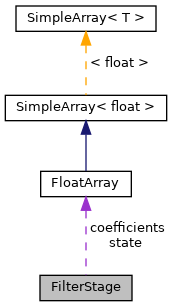OpenWareLaboratory
FilterStage Class Reference
Collaboration diagram for FilterStage:[legend]

## Public Member Functions

void copyCoefficients (FloatArray newCoefficients)

FilterStage (FloatArray co, FloatArray st)

FloatArray getCoefficients ()

FloatArray getState ()

void setAllPass (float fc, float q, float sr)

void setBandPass (float fc, float q, float sr)

void setHighPass (float fc, float q, float sr)

void setHighShelf (float fc, float gain, float sr)

void setLowPass (float fc, float q, float sr)

void setLowShelf (float fc, float gain, float sr)

void setNotch (float fc, float q, float sr)

void setPeak (float fc, float q, float gain, float sr)

## Static Public Member Functions

static void setAllPass (float *coefficients, float omega, float q)

static void setBandPass (float *coefficients, float omega, float q)

static void setHighPass (float *coefficients, float omega, float q)

static void setHighShelf (float *coefficients, float omega, float gain)

static void setLowPass (float *coefficients, float omega, float q)

static void setLowShelf (float *coefficients, float omega, float gain)

static void setNotch (float *coefficients, float omega, float q)

static void setPeak (float *coefficients, float omega, float q, float gain)

## Data Fields

FloatArray coefficients

FloatArray state

## Static Public Attributes

static constexpr float BESSEL_Q = 0.57735026919f

static constexpr float BUTTERWORTH_Q = M_SQRT1_2

static constexpr float SALLEN_KEY_Q = 0.5f

## Detailed Description

Definition at line 8 of file BiquadFilter.h.

## ◆ FilterStage()

 FilterStage::FilterStage ( FloatArray co, FloatArray st )
inline

Definition at line 16 of file BiquadFilter.h.

## ◆ copyCoefficients()

 void FilterStage::copyCoefficients ( FloatArray newCoefficients )
inline

Definition at line 47 of file BiquadFilter.h.

## ◆ getCoefficients()

 FloatArray FilterStage::getCoefficients ( )
inline

Definition at line 52 of file BiquadFilter.h.

References coefficients.

## ◆ getState()

 FloatArray FilterStage::getState ( )
inline

Definition at line 55 of file BiquadFilter.h.

References state.

## ◆ setAllPass() [1/2]

 static void FilterStage::setAllPass ( float * coefficients, float omega, float q )
inlinestatic

Definition at line 89 of file BiquadFilter.h.

References coefficients.

## ◆ setAllPass() [2/2]

 void FilterStage::setAllPass ( float fc, float q, float sr )
inline

Definition at line 30 of file BiquadFilter.h.

References coefficients, and M_PI.

## ◆ setBandPass() [1/2]

 static void FilterStage::setBandPass ( float * coefficients, float omega, float q )
inlinestatic

Definition at line 79 of file BiquadFilter.h.

References coefficients.

## ◆ setBandPass() [2/2]

 void FilterStage::setBandPass ( float fc, float q, float sr )
inline

Definition at line 26 of file BiquadFilter.h.

References coefficients, and M_PI.

## ◆ setHighPass() [1/2]

 static void FilterStage::setHighPass ( float * coefficients, float omega, float q )
inlinestatic

Definition at line 69 of file BiquadFilter.h.

References coefficients.

## ◆ setHighPass() [2/2]

 void FilterStage::setHighPass ( float fc, float q, float sr )
inline

Definition at line 22 of file BiquadFilter.h.

References coefficients, and M_PI.

## ◆ setHighShelf() [1/2]

 static void FilterStage::setHighShelf ( float * coefficients, float omega, float gain )
inlinestatic

Definition at line 152 of file BiquadFilter.h.

References coefficients, and M_SQRT2.

## ◆ setHighShelf() [2/2]

 void FilterStage::setHighShelf ( float fc, float gain, float sr )
inline

Definition at line 44 of file BiquadFilter.h.

References coefficients, and M_PI.

## ◆ setLowPass() [1/2]

 static void FilterStage::setLowPass ( float * coefficients, float omega, float q )
inlinestatic

Definition at line 59 of file BiquadFilter.h.

References coefficients.

## ◆ setLowPass() [2/2]

 void FilterStage::setLowPass ( float fc, float q, float sr )
inline

Definition at line 18 of file BiquadFilter.h.

References coefficients, and M_PI.

## ◆ setLowShelf() [1/2]

 static void FilterStage::setLowShelf ( float * coefficients, float omega, float gain )
inlinestatic

Definition at line 131 of file BiquadFilter.h.

References coefficients, and M_SQRT2.

## ◆ setLowShelf() [2/2]

 void FilterStage::setLowShelf ( float fc, float gain, float sr )
inline

Definition at line 41 of file BiquadFilter.h.

References coefficients, and M_PI.

## ◆ setNotch() [1/2]

 static void FilterStage::setNotch ( float * coefficients, float omega, float q )
inlinestatic

Definition at line 99 of file BiquadFilter.h.

References coefficients.

## ◆ setNotch() [2/2]

 void FilterStage::setNotch ( float fc, float q, float sr )
inline

Definition at line 34 of file BiquadFilter.h.

References coefficients, and M_PI.

## ◆ setPeak() [1/2]

 static void FilterStage::setPeak ( float * coefficients, float omega, float q, float gain )
inlinestatic

Definition at line 109 of file BiquadFilter.h.

References coefficients.

## ◆ setPeak() [2/2]

 void FilterStage::setPeak ( float fc, float q, float gain, float sr )
inline

Definition at line 38 of file BiquadFilter.h.

References coefficients, and M_PI.

## ◆ BESSEL_Q

 constexpr float FilterStage::BESSEL_Q = 0.57735026919f
staticconstexpr

Definition at line 12 of file BiquadFilter.h.

## ◆ BUTTERWORTH_Q

 constexpr float FilterStage::BUTTERWORTH_Q = M_SQRT1_2
staticconstexpr

Definition at line 14 of file BiquadFilter.h.

## ◆ coefficients

 FloatArray FilterStage::coefficients

Definition at line 10 of file BiquadFilter.h.

## ◆ SALLEN_KEY_Q

 constexpr float FilterStage::SALLEN_KEY_Q = 0.5f
staticconstexpr

Definition at line 13 of file BiquadFilter.h.

## ◆ state

 FloatArray FilterStage::state

Definition at line 11 of file BiquadFilter.h.

Referenced by getState().

The documentation for this class was generated from the following file: